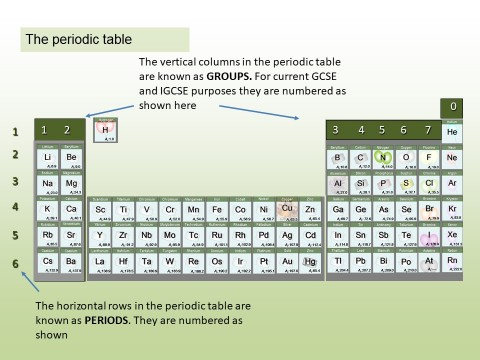ONLINE  ZOOM tution : Mob: +44 (0)7557331782 :  david@mychem.co.uk

# 1.37 - 1.39 Ionic bonding - transferring charge

#### 1.37 Electron transfer

A lightning strike contains billions of ions

Electrostatic forces are responsible for holding together the atoms in all substances. These forces are known as bonds, chemical bonds.

Chemical bonds are set up when electrons are transferred or shared between atoms. In this section we consider Ionic bonds . These form when atoms of metals transfer electrons to atoms of non-metals. The metal atoms lose electrons and become positive ions.  The non - metal atoms gain electrons and form negative ions.

Assumed background knowledge:### 1.18 - 1.21 The periodic table

1.18 Organising the Elements   Student...

#### Students should:

• 1.37 understand how ions are formed by electron loss or gain

Watch the video closely pausing where instructed. Write down your answers to the following questions.

1. How do positively charged ions form from atoms?
2. What are cations?
3. How do anions form from atoms?
4. What is the other word  used for outermost shell?
5. When atoms form ions what can you say about the arrangement of electrons in the outermost shell

#### 1.38 Activity 2. Know your ions.

These ions' charges cannot be worked out easily. You need to learn them!

#### Students should:

• 1.38 know the charges of common ions listed

You will have noted from the video that metal atoms tend to lose the electrons in their outermost electrons and form positive ions. The charge of the ion formed is equal to the number of electrons lost.

Non metal atoms gain electrons (when bonding with metals) and form negative ions. The non- metal atom gains enough electrons to complete its outermost shell

Use these ideas and the periodic table given to complete the following exercise.

1. Write out the symbols of the first 20 elements in order - as they appear on the periodic table.
3. From the information given in the video, write down charges on the ions.
4. See if you can work out the pattern and add it to your table.
Monatomic ions. This pattern of charges extends down the groups shown. You should learn the pattern

#### Students should:

• 1.39 write formulae for compounds formed between the ions listed

Watch the video closely pausing where instructed. Use the information given to work out the correct formulae for the following compounds:

1. lithium nitride
2. potassium oxide
3. zinc oxide
4. silver chloride
1. Li3
2. K2O
3. ZnO
4. AgCl

#### 1.3.9 A neat trick

A simple way to work out the formula of an ionic compound.

You can use this method to balance the charges and therefore work out the formula of any combination of positive and negative ions.

Note :

If the charge is 1- or 1+  we do not put the number 1 in the formula. For example : for sodium chloride we write NaCl rather than Na1Cl1

If the charges are  2+ and 2-  ( as in magnesium and oxygen) , the crossover method would give  us  Mg2O2  but we simplify this and write it as MgO.

So far we have considered only monatomic ions. These are ions formed when single atoms gain or lose electrons.

#### 1.3.9 Activity 4. Polyatomic ions

the formulae of five polyatomic ions and one monatomic ion to be learnt.

Polyatomic ions are ions which are made up of more than one atom . Each of these ions can exist as a single particle. Use the key and the formulae to match the images below to the formulae

You should learn these ions , their formula and charges.

#### 1.3.9 Activity 4. Polyatomic formulae

When writing formula involving polyatomic ions you need to be careful to use round brackets appropriately. The video here explains why.

Watch the video carefully and use it to note down the formulae of the following compounds :

** Work these out yourself :